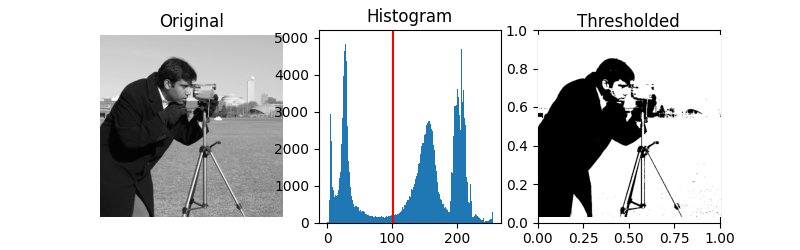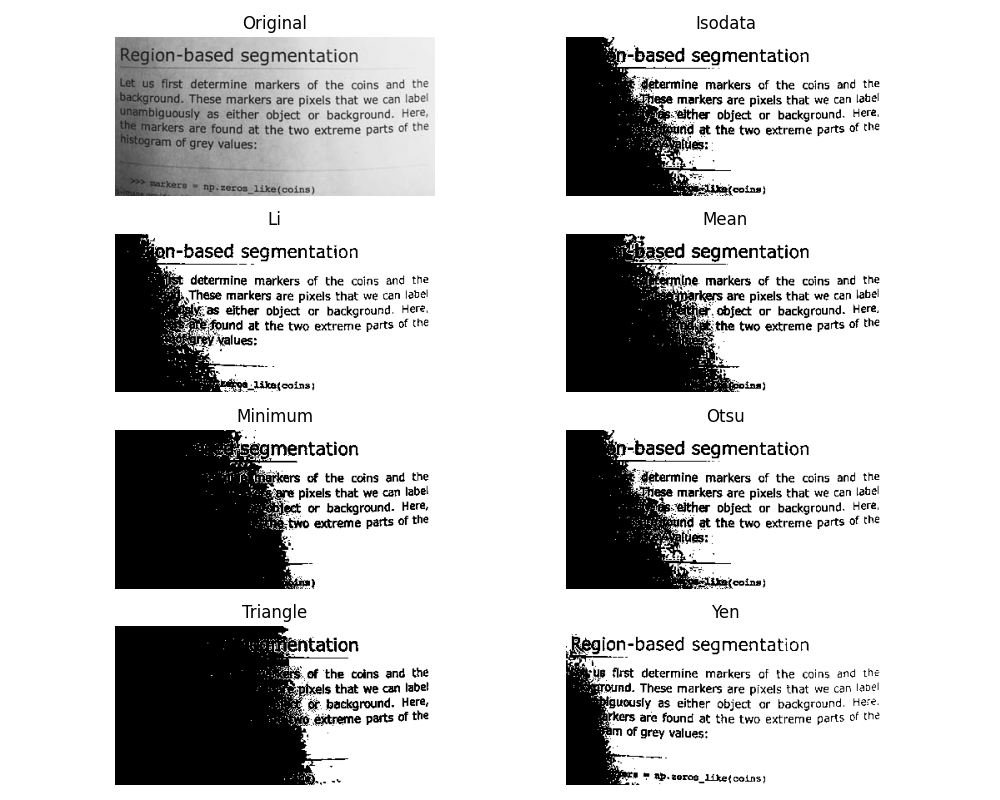# Thresholding#

Thresholding is used to create a binary image from a grayscale image .

A more comprehensive presentation on Thresholding

```import matplotlib.pyplot as plt
from skimage import data
from skimage.filters import threshold_otsu
```

We illustrate how to apply one of these thresholding algorithms. Otsu’s method  calculates an “optimal” threshold (marked by a red line in the histogram below) by maximizing the variance between two classes of pixels, which are separated by the threshold. Equivalently, this threshold minimizes the intra-class variance.

```image = data.camera()
thresh = threshold_otsu(image)
binary = image > thresh

fig, axes = plt.subplots(ncols=3, figsize=(8, 2.5))
ax = axes.ravel()
ax = plt.subplot(1, 3, 1)
ax = plt.subplot(1, 3, 2)
ax = plt.subplot(1, 3, 3, sharex=ax, sharey=ax)

ax.imshow(image, cmap=plt.cm.gray)
ax.set_title('Original')
ax.axis('off')

ax.hist(image.ravel(), bins=256)
ax.set_title('Histogram')
ax.axvline(thresh, color='r')

ax.imshow(binary, cmap=plt.cm.gray)
ax.set_title('Thresholded')
ax.axis('off')

plt.show()
``````/home/runner/work/scikit-image/scikit-image/doc/examples/segmentation/plot_thresholding.py:39: MatplotlibDeprecationWarning:

Auto-removal of overlapping axes is deprecated since 3.6 and will be removed two minor releases later; explicitly call ax.remove() as needed.
```

If you are not familiar with the details of the different algorithms and the underlying assumptions, it is often difficult to know which algorithm will give the best results. Therefore, Scikit-image includes a function to evaluate thresholding algorithms provided by the library. At a glance, you can select the best algorithm for your data without a deep understanding of their mechanisms.

```from skimage.filters import try_all_threshold

img = data.page()

fig, ax = try_all_threshold(img, figsize=(10, 8), verbose=False)
plt.show()
```Total running time of the script: ( 0 minutes 1.343 seconds)

Gallery generated by Sphinx-Gallery